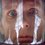# Irrationality and equations

The post by Kevin Chung reminded me of an interesting equation, proposed to me by a friend.

Problem. Prove that there no non-trivial rational solutions $a,b,c$ to the equation $a+b\sqrt{2}+c\sqrt{4}=0.$

Solution. If we multiplicate original equation by $\sqrt{2}$, we'll obtain two similar equations. Lets write them all down $\begin{cases} a+b\sqrt{2}+c\sqrt{4}=0 \\ a\sqrt{2}+b\sqrt{4}+2c=0 \\ a\sqrt{4}+2b+c\sqrt{2}=0 \end{cases}$

Now we may try to think backwards. Could possibly a system of linear equations with rational coefficients give an irrational solution? Applying Cramer's rule we see that it's not the case. However, our system clearly has a solution $(2,\sqrt{2},\sqrt{4})$. Which contradicts the assumption that $(a,b,c)$ are rational numbers (keep in mind that we throw trivial $(0,0,0)$ solution away).

Can you propose any generalizations? What features of the tuple $(1,\sqrt{2},\sqrt{4})$ does this relation imply? Have you noticed how this relation is similar to conditions of linear independence?Note by Nicolae Sapoval
5 years, 11 months ago

This discussion board is a place to discuss our Daily Challenges and the math and science related to those challenges. Explanations are more than just a solution — they should explain the steps and thinking strategies that you used to obtain the solution. Comments should further the discussion of math and science.

When posting on Brilliant:

• Use the emojis to react to an explanation, whether you're congratulating a job well done , or just really confused .
• Ask specific questions about the challenge or the steps in somebody's explanation. Well-posed questions can add a lot to the discussion, but posting "I don't understand!" doesn't help anyone.
• Try to contribute something new to the discussion, whether it is an extension, generalization or other idea related to the challenge.

MarkdownAppears as
*italics* or _italics_ italics
**bold** or __bold__ bold
- bulleted- list
• bulleted
• list
1. numbered2. list
1. numbered
2. list
Note: you must add a full line of space before and after lists for them to show up correctly
paragraph 1paragraph 2

paragraph 1

paragraph 2

[example link](https://brilliant.org)example link
> This is a quote
This is a quote
    # I indented these lines
# 4 spaces, and now they show
# up as a code block.

print "hello world"
# I indented these lines
# 4 spaces, and now they show
# up as a code block.

print "hello world"
MathAppears as
Remember to wrap math in $$ ... $$ or $ ... $ to ensure proper formatting.
2 \times 3 $2 \times 3$
2^{34} $2^{34}$
a_{i-1} $a_{i-1}$
\frac{2}{3} $\frac{2}{3}$
\sqrt{2} $\sqrt{2}$
\sum_{i=1}^3 $\sum_{i=1}^3$
\sin \theta $\sin \theta$
\boxed{123} $\boxed{123}$

Sort by:

What is Cramer's rule??

- 5 years, 8 months ago

It's one of the methods for solving systems of linear equations.. If you haven't studied matrix before, that'll be touch job for you. =3=

Google for that because I don't know how to explain that easily. T__T

- 5 years, 4 months ago

If I may provide a "better" solution...

We note that we are considering arbitrary elements of $\mathbb{Q}(\sqrt{2})$. Also note that the minimal polynomial of $\sqrt{2}$ over $\mathbb{Q}$ is $x^3-2$, which has degree 3. Thus, $[\mathbb{Q}(\sqrt{2}):\mathbb{Q}]=3$, so we have that $\mathbb{Q}(\sqrt{2})$ is a vector space over $\mathbb{Q}$ with basis $\{1,\sqrt{2},(\sqrt{2})^2\}$. Thus, there cannot be a nontrivial solution to $a+b\sqrt{2}+c\sqrt{4}=0$ because $\{1,\sqrt{2},(\sqrt{2})^2\}$ is linearly independent.

- 5 years, 11 months ago

Bravissimo, that is exactly what I expected -- a larger context! Any ideas how to broaden the result? Maybe some more general equations?

- 5 years, 11 months ago

I don't follow what you're saying. How would you like me to generalize?

- 5 years, 11 months ago

I think , there exist a very simple solution , Since $a+b\sqrt{2}+c\sqrt{4}=0 \implies a^3+2b^3+4c^3=6abc$ Also , WLOG we can assume $a,b,c \in \mathbb{Z}$ Now we must have $a$ even , this will then give $b$ even , and further going , by FMI(Fermat method of infinite descent , we get contradiction.

- 5 years, 11 months ago

Indeed very clever and beautiful solution!

- 5 years, 11 months ago